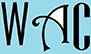## Geometry Question for Title 1 Schools: Perimeter & Length Question?

8) If the length of a rectangle is twice the width of it, what is the length if the perimeter is 30?

Perimeter is the sum of the sides of shape

Perimeter for rectangle “p” = 2 (length “l” + width “w”) to cover all 4 sides.

Since “l” is twice “w”, then l = 2w

p =  2 (l + w)

30 = 2 (2w + w)

30 = 2 (3w)

We have to make “w” subject of formula, so we first move 2 to the other side, then 3.  When you move to the other side, addition becomes subtraction, multiplication becomes division, and vice versa.

30/2 = 3w (2 was a multiplication at the other side)

15 = 3w

15/3 = w (3 multiplied w at the other side)

5 = w

If the width is 5, then the length must be 10.